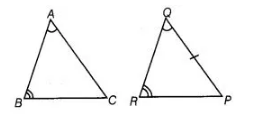# In ΔABC and ΔPQR,`
Question:

In ΔABC and ΔPQR, ∠A = ∠Q and ∠B = ∠R. Which side of  ΔPQR should be equal to side BC of ΔABC, so that the two triangles are congruent? Give

Solution:

We have given, in ΔABC and ΔPQR,

∠A = ∠Q and ∠B = ∠R

Since, two pairs of angles are equal in two triangles.

We know that, two triangles will be congruent by AAS rule, if two angles and the side of one triangle are equal to the two angles and the side of

other triangle.

∴ BC = RP